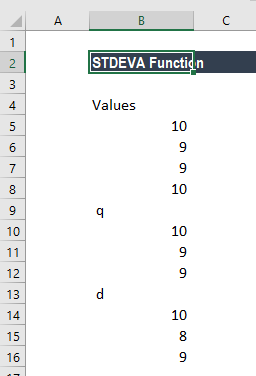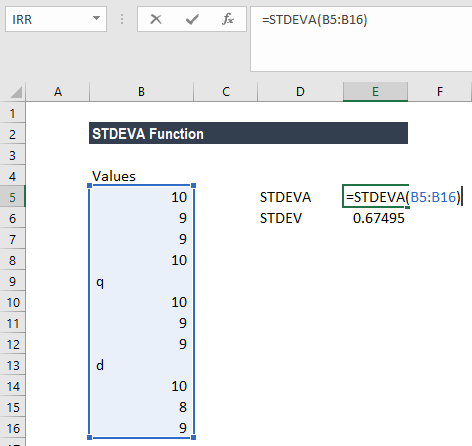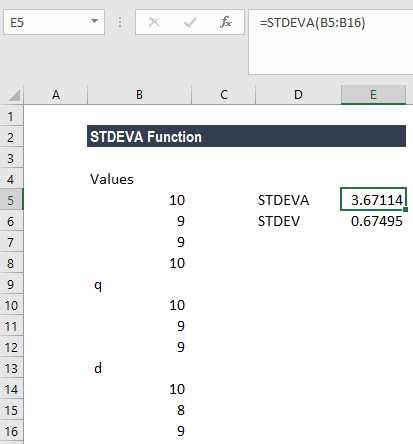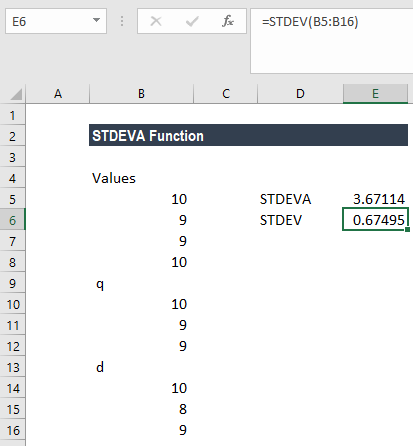# STDEVA Function

Get the standard deviation based on a sample

## What is the STDEVA Function?

The STDEVA Function is categorized under Excel Statistical functions. It will estimate the standard deviation based on a sample.

As a financial analyst, STDEVA can be useful in finding out the rate of return on an investment and measuring the investment’s volatility. If a stock is volatile, it will have a large standard deviation.  If a stock is stable, it will have a smaller standard deviation.

The biggest advantage of the STDEVA function is that it considers text provided in a given array as zero, which is not the case with other standard deviation functions such as STDEV.S, STDEV.P, etc.

### Formula

=STDEVA(number1,[number2],…)

The STDEVA function uses the following arguments:

1. Number1 (required argument) – This is the first number argument that corresponds to a sample of a population.
2. Number2 (optional argument) – This is a number argument that corresponds to a sample of a population.

#### A few notes on the arguments

1. The given arguments must provide at least two numeric values to the function.
2. The arguments should be between 1 and 255 numeric values, arrays of values, or references to cells containing numbers.
3. Arguments can either be numbers, names, arrays, or references that contain numbers.
4. The STDEVA function is used when we want to calculate the standard deviation of a sample of a population. If we are calculating the standard deviation of the entire population, we need to use the STDEVP or the STDEV.P function.

#### STDEVA and STDEV Functions

The STDEVA function is very similar to the STDEV function, in that both functions calculate the sample standard deviation of a supplied set of values.

However, they differ in one aspect. The difference arises when an array of values that contain text or logical values is supplied to the function. In such a case, STDEV ignores the text and logical values, whereas STDEVA assigns the value 0 to text and the values 1 or 0 to logical values.

### How to use the STDEVA Function in Excel?

To understand the uses of the STDEVA function, let’s consider an example:

#### Example

Suppose we are given the following data:The formula used is:We get the result below:When we apply the STDEVA function, it will evaluate the text as zero, which is not the case in the STDEV function. Due to this, the results we get under STDEV and STDEVA are different, as shown below:### Things to remember about the STDEVA Function

1. #DIV/0! error – Occurs if less than two numeric values are provided to the STDEVA function.
2. VALUE error – Occurs if any of the given value provided directly to the STDEVA function are text values that cannot be interpreted as numeric values.
3. STDEVA should be used when we need to evaluate text or logical values in the standard deviation calculation.
4. The function calculates standard deviation using the “n-1” method.
5. It assumes data as a sample. If data representations are for an entire population, the need to use STDEVP or STDEV.P.
6. Values are supplied as arguments. They can be supplied as actual numbers, ranges, arrays, or references that contain numbers.
7. STDEVA evaluates logical values and/or numbers in the references provided. To ignore the values, we need to use STDEV or STDEV.S.

Thanks for reading CFI’s guide to important Excel functions! By taking the time to learn and master these functions, you’ll significantly speed up your financial analysis. To learn more, check out these additional CFI resources:

• Excel Functions for Finance